# Data visualization with Pairplot Seaborn and Pandas

Data Visualization is the presentation of data in pictorial format. It is extremely important for Data Analysis, primarily because of the fantastic ecosystem of data-centric Python packages. And it helps to understand the data, however, complex it is, the significance of data by summarizing and presenting a huge amount of data in a simple and easy-to-understand format and helps communicate information clearly and effectively.Pandas and Seaborn is one of those packages and makes importing and analyzing data much easier. In this article, we will use Pandas and Pairplot Seaborn to analyze data.

## Pandas

Pandas Offer tools for cleaning and process your data. It is the most popular Python library that is used for data analysis. In pandas, a data table is called a dataframe.

Example 1:

## Python3

 `# Python code demonstrate creating  ` `   `  `import` `pandas as pd ` `   `  `# initialise data of lists. ` `data ``=` `{``'Name'``:[ ``'Mohe'` `, ``'Karnal'` `, ``'Yrik'` `, ``'jack'` `], ` `        ``'Age'``:[ ``30` `, ``21` `, ``29` `, ``28` `]} ` `   `  `# Create DataFrame ` `df ``=` `pd.DataFrame( data ) ` `   `  `# Print the output. ` `display(df)`

Output: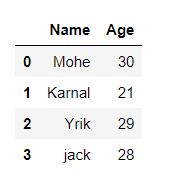Example 2: load the CSV data from the system and display it through pandas.

## Python3

 `# import module` `import` `pandas`   `# load the csv` `data ``=` `pandas.read_csv(``"nba.csv"``)`   `# show first 5 column` `data.head()`

Output: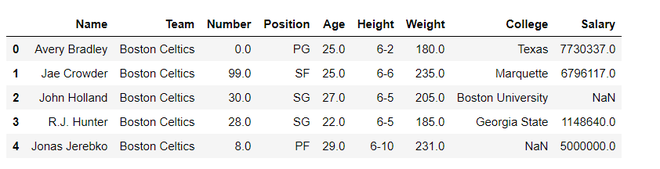## seaborn.pairplot()

To plot multiple pairwise bivariate distributions in a dataset, you can use the pairplot() function. This shows the relationship for (n, 2) combination of variable in a DataFrame as a matrix of plots and the diagonal plots are the univariate plots.

Syntax: seaborn.pairplot( data, \*\*kwargs )

Parameter:

data: Tidy (long-form) dataframe where each column is a variable and  each row is an observation.

hue: Variable in “data“ to map plot aspects to different colors.

palette: dict or seaborn color palette

{x, y}_vars: lists of variable names, optional

dropna: boolean, optional

Example 1:

## Python3

 `# importing packages ` `import` `seaborn ` `import` `matplotlib.pyplot as plt `   `# loading dataset using seaborn ` `df ``=` `seaborn.load_dataset(``'tips'``)`   `# pairplot with hue sex ` `seaborn.pairplot(df, hue ``=``'size'``) ` `plt.show() `

Output: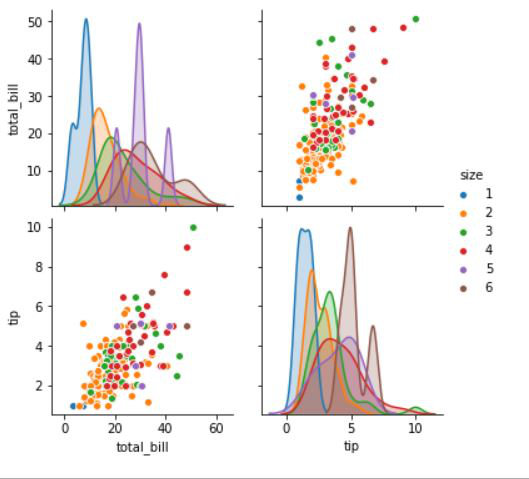## Data visualization with Pairplot Seaborn and pandas

We see how to create pandas dataframe and Pairplot. We will visualize data with pairplot using pandas

Example 1:

In this example, we will simply plot a pairplot with pandas data frame. Here we are simply loading nba.csv data and creating a dataframe and although passing as arguments in a pairplot.

## Python3

 `# importing packages ` `import` `seaborn ` `import` `pandas`   `# load the csv ` `data ``=` `pandas.read_csv(``"nba.csv"``) `   `# pairplot` `seaborn.pairplot(data)`

Output: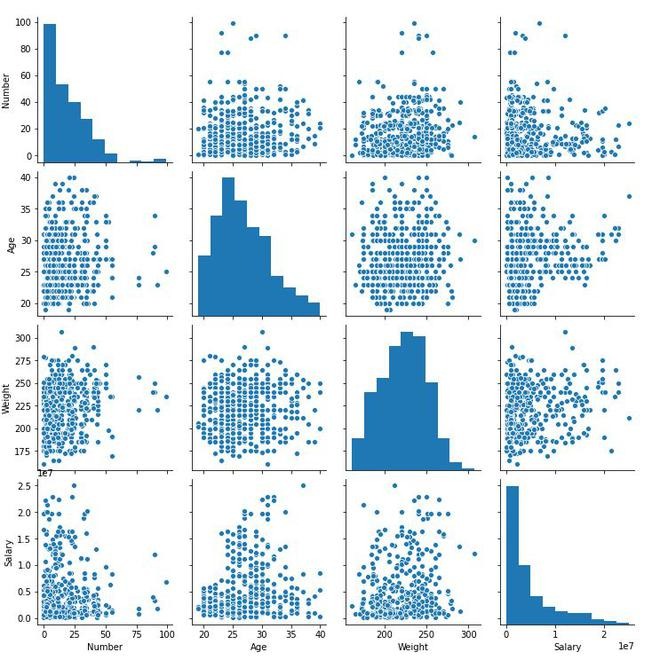Example 2:

In this example, we will be going to use hue attributes for the visualization of a specific column.

## Python3

 `# importing packages ` `import` `seaborn ` `import` `pandas`   `# load the csv ` `data ``=` `pandas.read_csv(``"nba.csv"``) ` `seaborn.pairplot(data.head(), hue ``=` `'Age'``)`

Output: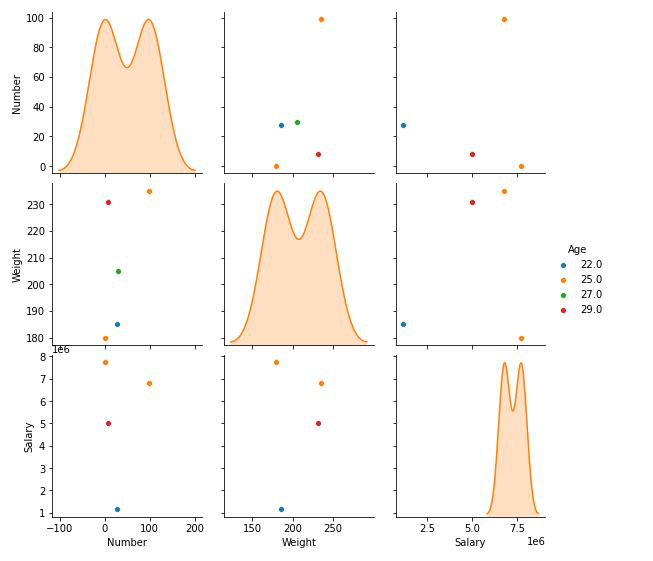Example 3:

In this example, we will pass the dictionaries of keyword arguments for bivariate plotting function(plot_kws and diag_kws)

## Python3

 `# importing packages ` `import` `seaborn ` `import` `pandas` `# load the csv ` `data ``=` `pandas.read_csv(``"nba.csv"``) `   `seaborn.pairplot(data, hue ``=` `'Age'``, diag_kind ``=` `'kde'``,` `             ``plot_kws ``=` `{``'edgecolor'``: ``'k'``}, size ``=` `4``)`

Output: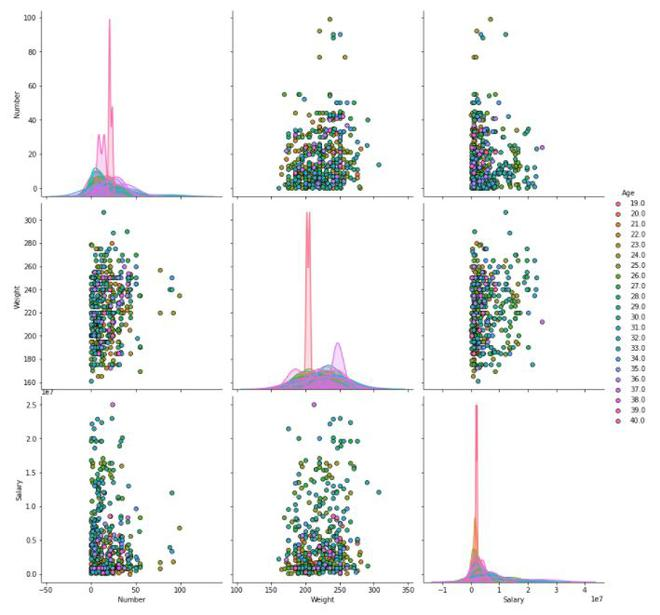Whether you're preparing for your first job interview or aiming to upskill in this ever-evolving tech landscape, GeeksforGeeks Courses are your key to success. We provide top-quality content at affordable prices, all geared towards accelerating your growth in a time-bound manner. Join the millions we've already empowered, and we're here to do the same for you. Don't miss out - check it out now!

Previous
Next